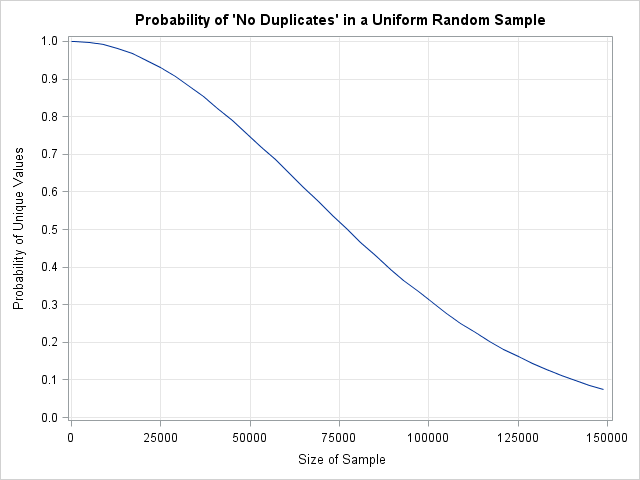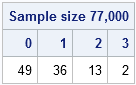As I wrote in my previous post, a SAS customer noticed that he was getting some duplicate values when he used the RAND function to generate a large number of random uniform values on the interval [0,1]. He wanted to know if this result indicates a bug in the RAND function. In my previous post, I demonstrated the following:

• In a long stream of uniformly distributed random numbers, you should expect to see duplicate values. If you do not see duplicate values, the stream is not very random.
• As the birthday matching problem shows, duplicates occur in samples that are much smaller than you might intuitively expect.

Let's apply these results to uniformly distributed uniform numbers on the interval (0,1). The SAS RAND function generates numbers with 32-bit accuracy, so there are about 232 distinct values that it will return in the interval (0,1). Let's use this fact to apply the "birthday matching problem" to uniform random values in (0,1). We want to compute the probability of a sample of size N having all unique values (no duplicates) when there are approximately 232 total values ("birthdays") to choose from. The following SAS/IML statements compute this probability for a samples of size 1–150,000:

```proc iml; K = 150000; /* max sample size */ M = 2##32; /* number of unique values */ i = 1:K; /* enumerate possible sample sizes */ iQ = 1 - (i-1)/M; /* individual probabilities */ Q = cuprod(iQ); /* probability of "no duplicates" for each sample size */```The graph at the left shows a plot of sample sizes versus the probability of having no duplicates in the sample. You can see that in a sample of size 77,000, the probability of having a duplicate is about 0.5. (The probability is 0.5 when the sample size is 77,163.) For random samples of size 150,000, the probability of a duplicate is almost 93%.

This result is an example of the "birthday matching problem," and it is counterintuitive. When I began looking at this problem, my intuition was that duplicates would appear when the size of the random sample got close to a million. After all, there are almost 232 (more than four billion) possible values that the RAND function can generate! But as the sample size grows, so does the number of previous values that the next value can match. As these results demonstrate, duplicate values are expected in a random uniform sample that is as small as 77,000. For a sample of size one million, you can expect about 116 duplicates.

To make sure that I haven't made a major mistake, the following SAS/IML statements simulate 100 samples with uniform random numbers. (The SAS/IML RANDGEN function, like the RAND function in Base SAS, calls the Mersenne-Twister algorithm.) Each sample is of size 77,000.

```K = 77000; /* sample size */ u = j(K, 100); /* each col will be a sample; create 100 samples */ call randseed(12345); call randgen(u, "Uniform"); /* fill with random uniform values */ NumDups = K - countunique(u, "col"); /* count number of dups in each sample */ call tabulate(vals, freq, NumDups); /* how many samples had 0, 1, 2, ... dups? */ print freq[label="Sample size 77,000" colname=(char(vals))];```The theory says that about half of the samples will contain duplicate values. The simulation shows that 49 out of the 100 samples do not contain duplicates, whereas 51 contain one or more duplicates. If you change the sample size (K) to 150,000 and rerun the program, you will see that the simulation also agrees with the theoretical result that about 93% of samples that large contain duplicates.

You can run the same experiment in other statistical computing languages and observe similar results. Derek O'Connor (2011) wrote a very nice article about duplicate values in random number streams in the MATLAB language. O'Connor concludes that duplicates are "not a deﬁciency in MATLAB’s ...random number generator..., but the inevitable result" of generating a long vector of random values. The documentation for the R language random number generators states that "most of the supplied uniform generators return... at most 2^32 distinct values and long runs will return duplicated values." In the R language you can use an expression such as sum(duplicated(runif(150000))) to examine the number of duplicate values.

In conclusion, it is not a bug to find duplicate values in a moderately sized sample of uniformly distributed values. The underlying probabilities (as encountered in the familiar "birthday matching problem") show that this behavior is correct. For samples of size 77,000 or more, duplicate values are expected.

ShareDistinguished Researcher in Computational Statistics

Rick Wicklin, PhD, is a distinguished researcher in computational statistics at SAS and is a principal developer of SAS/IML software. His areas of expertise include computational statistics, simulation, statistical graphics, and modern methods in statistical data analysis. Rick is author of the books Statistical Programming with SAS/IML Software and Simulating Data with SAS.

1.2.•